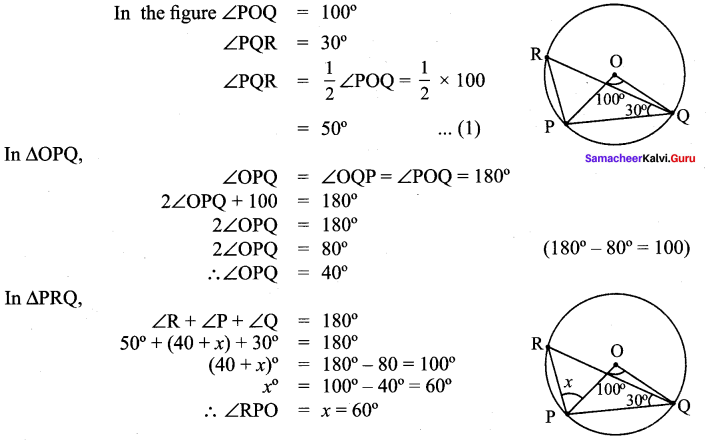## Tamilnadu Samacheer Kalvi 9th Maths Solutions Chapter 4 Geometry Ex 4.4

Question 1.
Find the value of x in the given figure.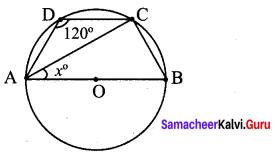Solution:
∠ABC = 180° – 120° = 60°
∠BCA = 90°
∴ x = ∠BAC = 180°- (90° + 60°) = 30°

Question 2.
In the given figure, AC is the diameter of the circle with centre O. If ∠ADE = 30°; ∠DAC = 35° and ∠CAB = 40°.
Find
(i) ∠ACD
(ii) ∠ACB
(iii) ∠DAE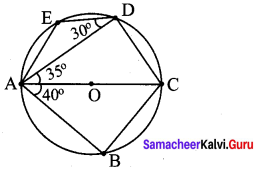Solution:
(i) ∠ACD = 180°- (90° + 35°) = 180°- 125° = 55°
(ii) ∠ACB = 180°- (90°+ 40°)= 180° – 130° = 50°
∠CAE = 180° – 120° = 60°
∴ ∠DAE = 60°- 35° = 25°

Question 3.
Find all the angles of the given cyclic quadrilateral ABCD in the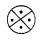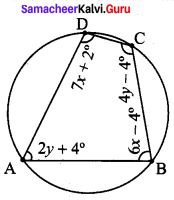Solution: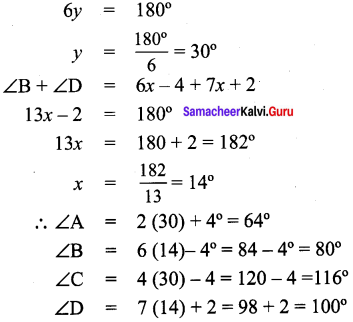Question 4.
In the given figure, ABCD is a cyclic quadrilateral where diagonals intersects at P such that ∠DBC = 40° and ∠BAC = 60° find
(ii) ∠BCD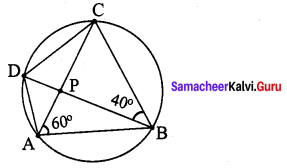Solution: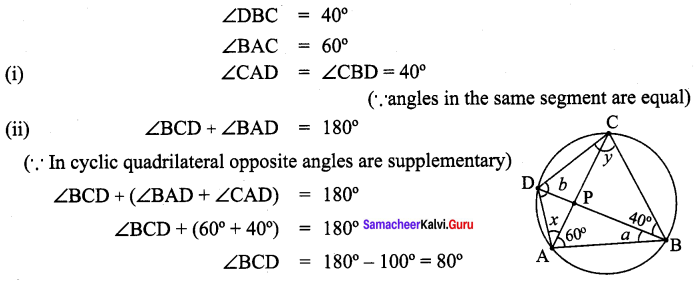Question 5.
In the given figure, AB and CD are the parallel chords of a circle with centre O. Such that AB = 8 cm and CD = 6 cm. If OM ⊥ AB and OL ⊥ CD distance between LM is 7 cm. Find the radius of the circle?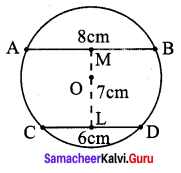Solution:
In the figure LM = 7 cm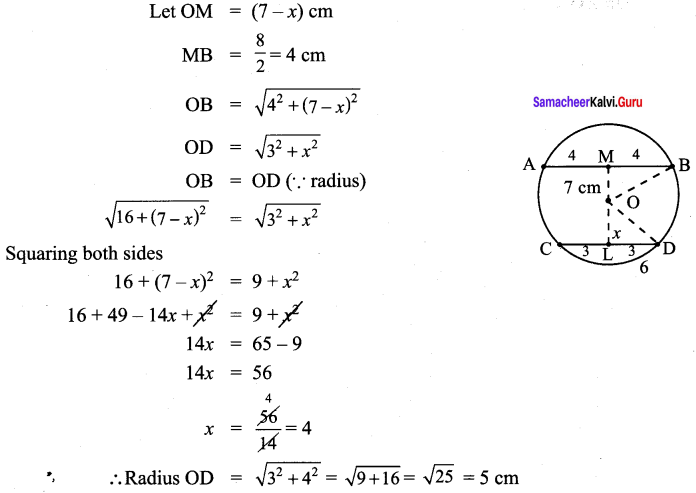Question 6.
The arch of a bridge has dimensions as shown, where the arch measure 2 m at its highest point and its width is 6 m. What is the radius of the circle that contains the arch?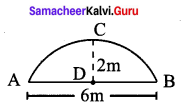Solution: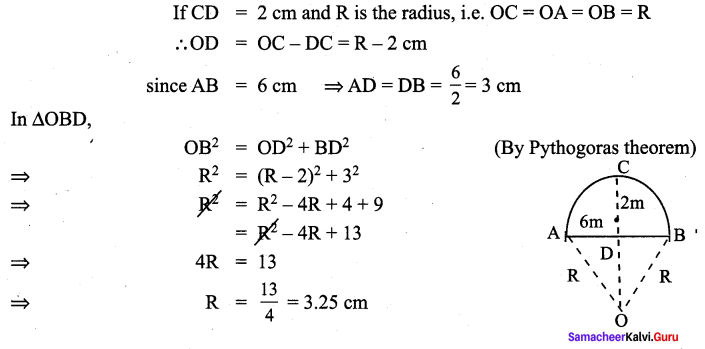Question 7.
In figure ∠ABC = 120°, where A,B and C are points on the circle with centre O. Find ∠OAC ?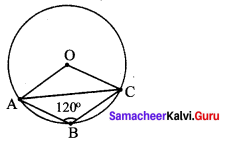Solution: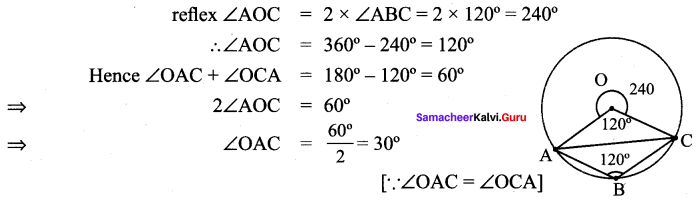Question 8.
A school wants to conduct tree plantation programme. For this a teacher allotted a circle of radius 6 m ground to nineth standard students for planting sapplings. Four students plant trees at the points A, B, C and D as shown in figure. Here AB = 8 m, CD = 10 m and AB ⊥ CD. If another student places a flower pot at the point P, the intersection of AB and CD, then find the distance from the centre to P.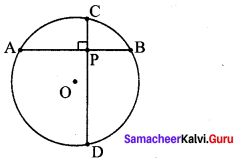Solution: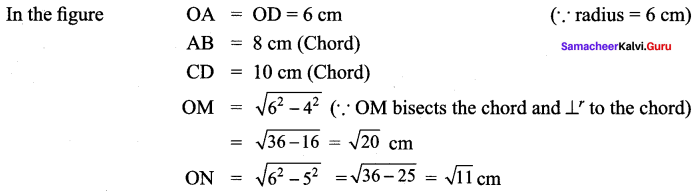ONPM is a rectangle with all the angles 90° and with length $$\sqrt{20}$$ cm, breadth $$\sqrt{11}$$ cm.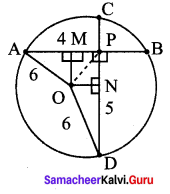We need to find OP which is the diagonal of the rectangle ONPM.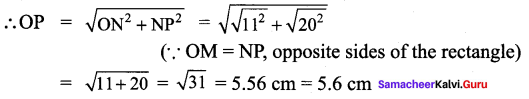Question 9.
In the given figure, ∠POQ = 100° and ∠PQR = 30°, then find ∠RPO.
Solution: